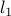# Model Selection Through Sparse Maximum Likelihood Estimation for Multivariate Gaussian or Binary Data

• Authors: O. Banerjee, L. El Ghaoui, A. d'Aspremont.

• Status: Journal of Machine Learning Research, 9(Mar):485–516, 2008.

• Abstract: We consider the problem of estimating the parameters of a Gaussian or binary distribution in such a way that the resulting undirected graphical model is sparse. Our approach is to solve a maximum likelihood problem with an added-norm penalty term. The problem as formulated is convex but the memory requirements and complexity of existing interior point methods are prohibitive for problems with more than tens of nodes. We present two new algorithms for solving problems with at least a thousand nodes in the Gaussian case. Our first algorithm uses block coordinate descent, and can be interpreted as recursive-norm penalized regression. Our second algorithm, based on Nesterov's first order method, yields a complexity estimate with a better dependence on problem size than existing interior point methods. Using a log determinant relaxation of the log partition function (Wainwright & Jordan (2006)), we show that these same algorithms can be used to solve an approximate sparse maximum likelihood problem for the binary case. We test our algorithms on synthetic data, as well as on gene expression and senate voting records data.

• Bibtex reference:

```@article{BEA:08,
Author = {O.Banerjee and L. {El Ghaoui} and A. {d'Aspremont}},
Journal = {Journal of Machine Learning Research},
Pages = {485-516},
Title = {Model Selection Through Sparse Maximum Likelihood
Estimation for Multivariate Gaussian or Binary Data},
Volume = {9},
Month = {March},
Year = {2008}}
```